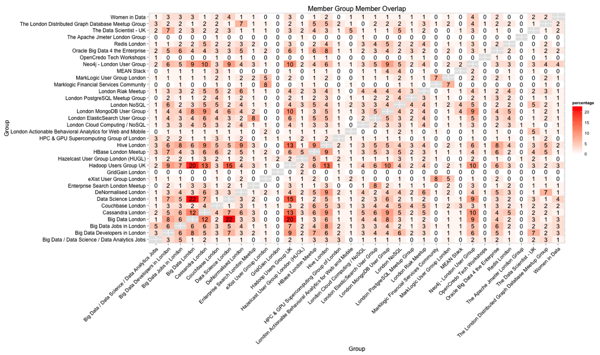# Neo4j/R: Analysing London NoSQL meetup membership

In my spare time I’ve been working on a Neo4j application that runs on tops of meetup.com’s API and Nicole recently showed me how I could wire up some of the queries to use her Rneo4j library:

The query used in that visualisation shows the number of members that overlap between each pair of groups but a more interesting query is the one which shows the % overlap between groups based on the unique members across the groups.

The query is a bit more complicated than the original:

``````MATCH (group1:Group), (group2:Group)
OPTIONAL MATCH (group1)<-[:MEMBER_OF]-()-[:MEMBER_OF]->(group2)

WITH group1, group2, COUNT(*) as commonMembers
MATCH (group1)<-[:MEMBER_OF]-(group1Member)

WITH group1, group2, commonMembers, COLLECT(id(group1Member)) AS group1Members
MATCH (group2)<-[:MEMBER_OF]-(group2Member)

WITH group1, group2, commonMembers, group1Members, COLLECT(id(group2Member)) AS group2Members
WITH group1, group2, commonMembers, group1Members, group2Members

UNWIND(group1Members + group2Members) AS combinedMember
WITH DISTINCT group1, group2, commonMembers, combinedMember

WITH group1, group2, commonMembers, COUNT(combinedMember) AS combinedMembers

RETURN group1.name, group2.name, toInt(round(100.0 * commonMembers / combinedMembers)) AS percentage
ORDER BY group1.name, group1.name``````

The next step is to wire that up to use Rneo4j and ggplot2. First we’ll get the libraries installed and loaded:

``````install.packages("devtools")
devtools::install_github("nicolewhite/Rneo4j")
install.packages("ggplot2")

library(Rneo4j)
library(ggplot2)``````

And now we’ll execute the query and create a chart from the results:

``````graph = startGraph("http://localhost:7474/db/data/")

query = "MATCH (group1:Group), (group2:Group)
WHERE group1 <> group2
OPTIONAL MATCH p = (group1)<-[:MEMBER_OF]-()-[:MEMBER_OF]->(group2)
WITH group1, group2, COLLECT(p) AS paths
RETURN group1.name, group2.name, LENGTH(paths) as commonMembers
ORDER BY group1.name, group2.name"

group_overlap = cypher(graph, query)

ggplot(group_overlap, aes(x=group1.name, y=group2.name, fill=commonMembers)) +
geom_bin2d() +
geom_text(aes(label = commonMembers)) +
labs(x= "Group", y="Group", title="Member Group Member Overlap") +
theme(axis.text = element_text(size = 12, color = "black"),
axis.title = element_text(size = 14, color = "black"),
plot.title = element_text(size = 16, color = "black"),
axis.text.x = element_text(angle = 45, vjust = 1, hjust = 1))

// as percentage

query = "MATCH (group1:Group), (group2:Group)
WHERE group1 <> group2
OPTIONAL MATCH path = (group1)<-[:MEMBER_OF]-()-[:MEMBER_OF]->(group2)

WITH group1, group2, COLLECT(path) AS paths

WITH group1, group2, LENGTH(paths) as commonMembers
MATCH (group1)<-[:MEMBER_OF]-(group1Member)

WITH group1, group2, commonMembers, COLLECT(id(group1Member)) AS group1Members
MATCH (group2)<-[:MEMBER_OF]-(group2Member)

WITH group1, group2, commonMembers, group1Members, COLLECT(id(group2Member)) AS group2Members
WITH group1, group2, commonMembers, group1Members, group2Members

UNWIND(group1Members + group2Members) AS combinedMember
WITH DISTINCT group1, group2, commonMembers, combinedMember

WITH group1, group2, commonMembers, COUNT(combinedMember) AS combinedMembers

RETURN group1.name, group2.name, toInt(round(100.0 * commonMembers / combinedMembers)) AS percentage

ORDER BY group1.name, group1.name"

group_overlap = cypher(graph, query)

ggplot(group_overlap, aes(x=group1.name, y=group2.name, fill=percentage)) +
geom_bin2d() +
geom_text(aes(label = percentage)) +
labs(x= "Group", y="Group", title="Member Group Member Overlap") +